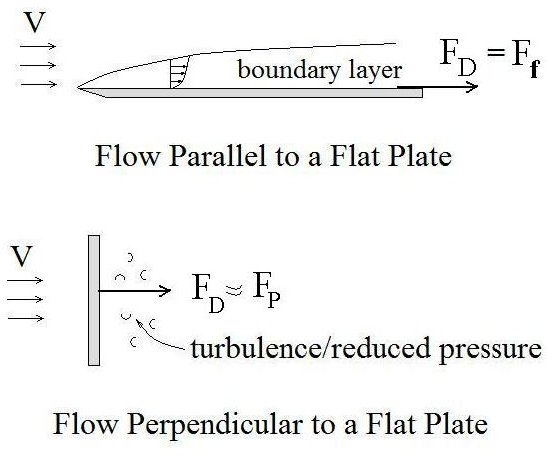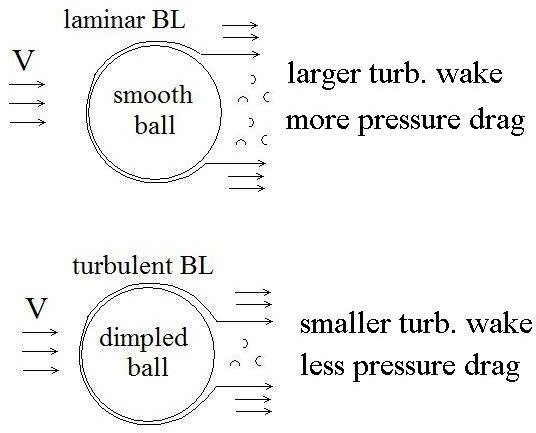# Why Golf Ball Dimples Make It Go Further: Less Air Resistance Due to Less Pressure Drag

## Introduction

Would you believe that, if both are hit with exactly the same force by a golf club, a golf ball with dimples will go much farther than a completely smooth golf ball of the same size and weight? Indeed it will, and that’s the reason that all golf balls in use today have dimpled surfaces. See ‘Drag Force for Fluid Flow Past an Immersed Object,’ for details of the general drag force equation, which relates drag force to velocity, fluid properties, object size and shape, and drag coefficient for fluid flow past any immersed object. This article zeros in on a ball moving through air, showing how golf ball dimples result in a lower drag coefficient, less air resistance, and less drag force. As a result the ball goes farther.

## A Bit of Golf Ball History

When a game much like the current game of golf was played in Scotland several centuries ago, balls with a smooth surface were used. Over the years, players noticed that old golf balls that had been nicked and marred by being hit with a golf club traveled farther than new, smooth, unblemished golf balls. Finally, in 1908, an Englishman, William Taylor, is credited with first manufacturing a golf ball having a dimpled surface. In time that design took over and golf ball dimples are present on any golf ball you see in use today. Following are some details of why that took place.

## Friction Drag and Pressure DragIn general, for an object moving through air, the air resistance/drag force due to the fluid flow past the object will be made up of two components, friction drag (based on a friction drag coefficient) and pressure drag (based on a pressure drag coefficient). The friction drag is due to friction between the solid surface and a thin layer of fluid adjacent to the surface called a boundary layer. The pressure drag is due to reduced pressure in a turbulent wake behind the solid object. For flow parallel to a flat surface, the drag force is essentially all friction drag. For flow perpendicular to a flat plate (like a billboard), the drag force is almost all pressure drag. This is shown in the diagram at the left.

## Fluid Flow Around and Air Resistance on a Sphere (Ball)

For flow around a ball (or a ball moving through a fluid), the air resistance will be due to both friction drag and pressure drag.There will be a boundary layer (and the resulting friction drag) on the front part of the ball. There will also be a turbulent wake behind the ball resulting in reduced pressure behind the ball and hence a drag force due to higher pressure in front of the ball than behind it.

Now, let’s consider a few useful facts about boundary layers. The flow in a boundary layer can be either laminar or turbulent. If the leading part of the surface is smooth then the first part of the boundary layer will be laminar and it will become turbulent at some point if the surface is long enough. If, however, the leading part of the surface is rough, then the entire boundary layer will be turbulent. Now the most interesting fact of all: A turbulent boundary layer will remain attached to the surface farther around to the back of the ball than a laminar boundary layer will. This results in a smaller turbulent wake behind the ball and less pressure drag. See the diagram at the right as an illustration of this effect. The frictional drag is greater with a turbulent boundary layer than with a laminar boundary layer, but the frictional drag is much smaller than the pressure drag for flow around a sphere, so the pressure drag effects are predominant.

So, you see, fluid mechanics can explain why a dimpled golf ball goes farther!

## Summary

Golf ball dimples are used on all golf balls because a dimpled golf ball has less air resistance than a smooth ball of the same size and weight. This means less drag force and the ball going farther. The lower air resistance is because the golf ball dimples cause a turbulent boundary layer that stays attached farther around to the back of the ball, making a smaller turbulent wake , a smaller pressure drag coefficient, and less pressure drag force on the ball.